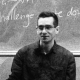## Symmetric Spaces and the Tenfold Way

I gave a talk about symmetric spaces and the tenfold way in Nicohl Furey’s series Algebra, Particles and Quantum Theory on Monday May 15, 2023. This talk was a followup to an earlier talk, also about the tenfold way.

You can see the slides of my new talk here. They have material on category theory that I didn’t get around to talking about.

Symmetric spaces and the tenfold way

Abstract: The tenfold way has many manifestations. It began as a tenfold classification of states of matter based on their behavior under time reversal and charge conjugation. Mathematically, it relies on the fact that there are ten super division algebras and ten kinds of Clifford algebras, where two Clifford algebras are of the same kind if they have equivalent super-categories of super-representations. But Cartan also showed that there are ten infinite families of compact symmetric spaces! After explaining symmetric spaces, we show how they arise naturally from forgetful functors between categories of representations of Clifford algebras.

The final upshot is this:

Let the Clifford algebra$\mathrm{Cliff}_{n}$ be the free real or complex algebra on n anticommuting square roots of -1. Let$F \colon \mathsf{Rep}(\mathrm{Cliff}_{n}) \to \mathsf{Rep}(\mathrm{Cliff}_{n-1})$

be the forgetful functor between representation categories. Then the essential fibers of this functor are disjoint unions of compact symmetric spaces. Moreover we get all the compact symmetric spaces in the 10 infinite families this way!

(There are also finitely many exceptional ones, which all arise from the octonions.)### 6 Responses to Symmetric Spaces and the Tenfold Way

1.David Roberts says:

It seems to me that it’s perfectly reasonable to have a disconnected compact symmetric space, since the definition doesn’t rely on connectedness, though it is included. The important thing is that for each point there is an involution fixing that point and acting as -1 on the tangent space there.

Clearly for the purposes of classification, getting the connected ones is enough. And if I’m not mistaken, each connected component of one of these “disconnected compact symmetric spaces” should be a symmetric space, too.

In the slides, the example where the essential fibre$F^{-1}(\mathbb{H}^n)$ is a disjoint union is where one has a two-parameter family, with the second parameter can be taken to have a finite range. I presume this is true for the other cases where one has these two parameter families (namely the real and complex Grassmannians)? One might make an argument that among the ten infinite families, they really should only have one parameter, just that three of those families consist of disconnected symmetric spaces (obviously with finitely many components).

•John Baez says:

It is indeed perfectly possible to have a disconnected symmetric space, but the usual definition requires connectedness so I didn’t want to mess with that in this talk. A really nice abstract definition of symmetric space would be ‘involutory quandle object in the category of smooth manifolds’, but then to recover Cartan’s classification we need to impose conditions like compactness, preservation of a Riemannian metric by the quandle operation$x \triangleright -,$ a condition saying that each point$x$ is an isolated fixed point of$x \triangleright -,$ and even more (some ‘irreducibility’ and simply-connectedness properties I didn’t want to talk about).

Anyway, all your guesses are right. There are three two-parameter families of compact symmetric spaces, the Grassmannians, ultimately because three Morita equivalence classes of Clifford algebras are not Morita equivalent to division algebras (whose representations are classified by a single natural number). Let me talk about the real case for simplicity. If you take the manifold of all subspaces of$\mathbb{R}^n$, it is a disjoint union of Grassmannians, but the whole thing is a quandle object, because it makes sense to reflect any subspace$W$ of$\mathbb{R}^n$ across any subspace$V,$ by applying the transformation that preserves$V$ and multiplies vectors orthogonal to$V$ by -1. So, it’s very nice—it just happens to be disconnected, as you noted.

2.Sir Lleb says:

•John Baez says:

Yes, and I should get the video and put it on YouTube at some point. I’ll announce that on this page when it happens.

•John Baez says:

Okay, I’ve added a video of the talk to this post!

•Sir Lleb says:

Thank you, much appreciated.

This site uses Akismet to reduce spam. Learn how your comment data is processed.